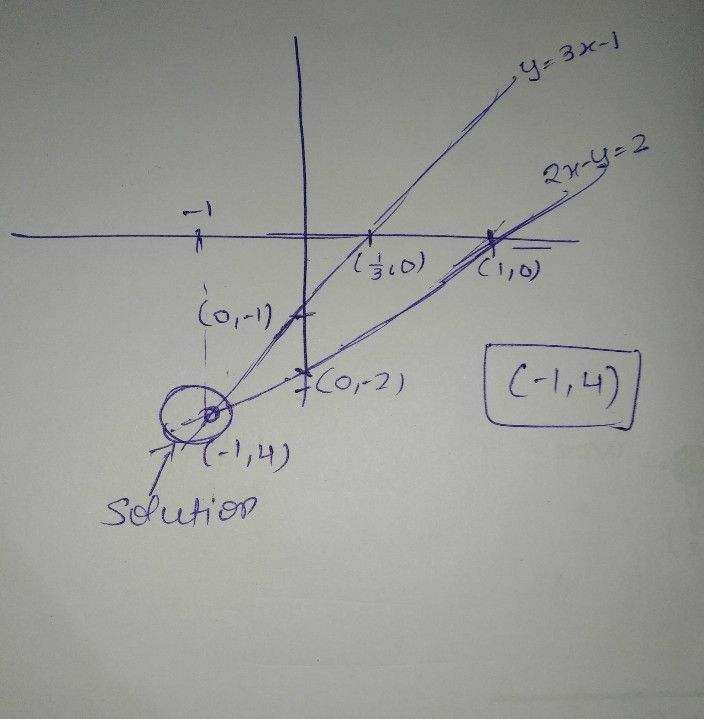Symbol
Problem$b$ $y=3x-1$ and $2x-y=2$ $y$ $x$